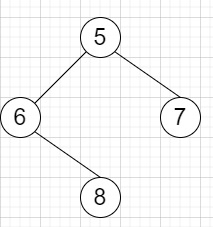# Construct String from Binary Tree in Python

Suppose we have a binary tree we have to make a string consists of parenthesis and integers from a binary tree with the preorder traversing way. A null node will be represented by empty parenthesis pair "()". And we need to omit all the empty parenthesis pairs that don't affect the one-to-one mapping relationship between the string and the original binary tree.

So, if the input is likethen the output will be 5(6()(8))(7)

To solve this, we will follow these steps −

• ans := blank string
• Define a function pot(). This will take node, s
• if is null, then
• return blank string
• if if left or right of node is null, then
• return node.data
• ss := node.data
• if node.left is not null, then
• ss := ss concatenate '(' concatenate pot(node.left, blank string) concatenate ')'
• otherwise,
• ss := ss concatenate '()'
• if node.right is not null, then
• ss := ss concatenate '(' concatenate pot(node.right, blank string) concatenate ')'
• return ss
• From the main method do the following −
• return pot(t, blank string)

Let us see the following implementation to get better understanding −

## Example

Live Demo

class TreeNode:
def __init__(self, data, left = None, right = None):
self.data = data
self.left = left
self.right = right
def insert(temp,data):
que = []
que.append(temp)
while (len(que)):
temp = que
que.pop(0)
if (not temp.left):
if data is not None:
temp.left = TreeNode(data)
else:
temp.left = TreeNode(0)
break
else:
que.append(temp.left)
if (not temp.right):
if data is not None:
temp.right = TreeNode(data)
else:
temp.right = TreeNode(0)
break
else:
que.append(temp.right)
def make_tree(elements):
Tree = TreeNode(elements)
for element in elements[1:]:
insert(Tree, element)
return Tree
class Solution:
def tree2str(self, t: TreeNode):
ans = ''
def pot(node, s):
if node == None or node.data == 0:
return ''
if node.left == node.right == None:
return str(node.data)
ss = str(node.data)
if node.left != None:
ss = ss + '(' + pot(node.left, '') + ')'
else:
ss = ss + '()'
if node.right != None:
ss = ss + '(' + pot(node.right, '') + ')'
return ss
return pot(t, '')
ob = Solution()
root = make_tree([5,6,7,None,8])
print(ob.tree2str(root))

## Input

[5,6,7,None,8]

## Output

5(6()(8))(7)